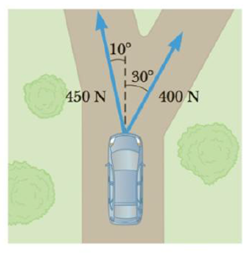Chapter 4, Problem 12P

Chapter
Section
Textbook Problem

Two forces are applied to a car in an effort to move it, as shown in Figure P4.12. (a) What is the resultant vector of these two forces? (b) If the car has a mass of 3 000 kg, what acceleration does it have? Ignore friction.Figure P4.12

(a)

To determine
The resultant vector of the forces.

Explanation

Section 1:

Explanation:

Given info: The magnitude of the two forces are F1 = 400 N and F2 = 450 N. The angle of inclination of F1 and F2 are marked in the figure. The mass of the car is 300 kg.

The forces along the x and y directions are given in the figure below.

The total force along the x-axis is,

Fx=F1sin30°F2sin10°

Substitute 400 N for F1 and 400 N for F2 in the above expression.

Fx=(400N)(0.5)(450N)(0.174)=121.86N

The fifference between the two forces is taken because the directions are opposite.

The total force along the y-axis is,

Fy=F1cos30°+F2cos10°

Substitute 400 N for F1 and 400 N for F2 in the above expression.

Fy=(400N)(0.866)+(450N)(0

(b)

To determine
The acceleration of the car.

Still sussing out bartleby?

Check out a sample textbook solution.

See a sample solution

The Solution to Your Study Problems

Bartleby provides explanations to thousands of textbook problems written by our experts, many with advanced degrees!

Get Started

Find more solutions based on key concepts